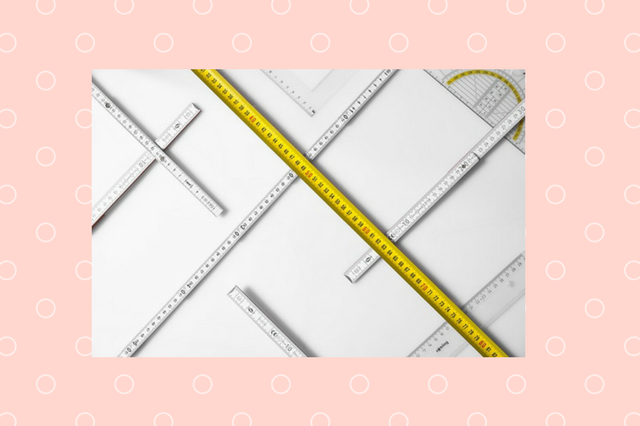Measurement

Year 1 Measurement Worksheets are available here.

The key concepts to know in Year 1 about measurement are as follows

• The standard units to measure length and height in any direction are centimeters(cm) and meters(m).
• The standard units to measure mass or weight are  kilograms(kg)and grams(g).
• The standard unit  to measure temperature is Centigrade (°C).
•  The standard units to measure capacity  are liters(L)and milli liters (ml).
• In order to measure thess units we use rulers, scales, thermometers and measuring vessels for different things.

Star fact:

1. There are 100 centimeters(cm) in 1 metre (m).i.e 100 cm = 1 m.
2.  There are 1000 milliliter (ml) in 1 L i.e 1000 ml=1 L.